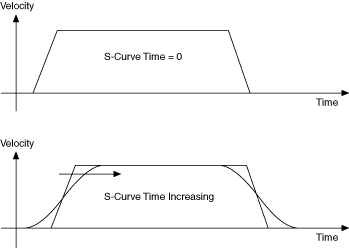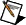# S Curve Time

Adjusting the s-curve time smooths the acceleration and deceleration portions of a motion profile, resulting in less abrupt transitions from starting a move to acceleration, acceleration to constant velocity, constant velocity to deceleration, and deceleration to stop. Using s-curve acceleration limits the jerk in a motion control system.

Officially, jerk is defined as the derivative of acceleration (change of acceleration per unit time) and is measured in units of counts (steps)/s3. This function, however, allows you to load s-curve time in update sample periods rather than have to deal with the obscure units of jerk.

With the default s-curve time of one (1) sample period, there is virtually no effect on the motion profile, and the standard trapezoidal trajectory is executed. As s-curve time increases, the smoothing affect on the acceleration and deceleration portions of the motion profile increase, as shown in the following figure. Large values of s-curve time can override the programmed values of acceleration and deceleration by sufficiently smoothing the profile such that the acceleration and deceleration slopes are never reached.Note  A large s-curve values makes the time to accelerate to the commanded velocity longer. Therefore, the time it takes to reach the target position is also longer. If the s-curve value is too large, the value is ignored and full acceleration is used. This happens when the acceleration and deceleration become too long to perform smoothly.